Reach Us+44-7482-875032
New Method for Homogeneous Smoluchowski Coagulation Equation | OMICS International
Journal of Applied & Computational Mathematics
All submissions of the EM system will be redirected to Online Manuscript Submission System. Authors are requested to submit articles directly to Online Manuscript Submission System of respective journal.

# New Method for Homogeneous Smoluchowski Coagulation Equation

Sadri K* and Ebrahimi H

Department of Mathematics, Rasht Branch, Islamic Azad University, P.O. Box.41335-3516, Rasht, Iran

*Corresponding Author:
Sadri K
Department of Mathematics, Rasht Branch
Islamic Azad University, P.O. Box.41335-3516, Rasht, Iran
Tel: 982147911
E-mail: [email protected]

Received June 19, 2015; Accepted July 04, 2015; Published July 10, 2015

Citation: Sadri K, Ebrahimi H (2015) New Method for Homogeneous Smoluchowski Coagulation Equation. J Appl Computat Math 4: 233. doi:10.4172/2168-9679.1000233

Copyright: © 2015 Sadri K, et al. This is an open-access article distributed under the terms of the Creative Commons Attribution License, which permits unrestricted use, distribution, and reproduction in any medium, provided the original author and source are credited.

Visit for more related articles at Journal of Applied & Computational Mathematics

#### Abstract

In this paper, variational iteration method is employed to solve the homogenous Smoluchowski coagulation
equation. The intervals of validity of the solutions will be extended by using Pade approximation. Error will be decrease, as it is expected. The numerical results show the effectiveness and the simplicity of the methods.

#### Keywords

Variational iteration method; Pade approximation; Homogenous Smoluchowski coagulation equation; Lagrange multiplier

#### Introduction

Variation iteration method (VIM) has been proposed by Ji-Huan He, in 1998, and has been applied to solve many linear and nonlinear functional equations. There are many research documents, in the literature, for application of VIM to solve different functional equations, such as [1-3].

Let's consider the following non-linear functional equation,

L(u(t)) + N(u(t)) + g(t) =0,

Where L, N and g(t) are a linear, a non-linear operator, and a known analytic function, respectively. In this method, a correction functional including a general Lagrange multiplier, will be constructed as follows,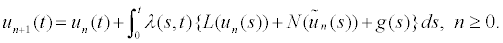Where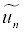is restricted variations, i.e.,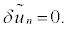. Lagrange multiplier can be identify optimally via the variation theory. An iterative formula, for computing the sequence of the approximations, will be obtained as soon as the Lagrange multiplier is determined. The successive approximations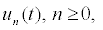of u(t) will be obtained by selection an initial approximation of the solution, u0. Initial and boundary conditions must be satisfied by initial approximation, u0. Iterative formula is constructed as follows;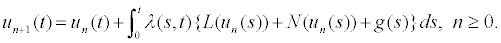(3)

Exact solution will be determined as the following limit,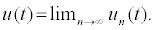(4)

#### Pade Approximation

The series solution obtained by VIM has a small region of convergence. To extend the region of convergence, Pade approximation will be helpful. Pade approximation of a function is given by the ratio of two polynomials . The coefficients of the polynomials in the numerator and denominator are determined by using the coefficients in the Taylor series expansion of the function. The Pade approximation of a function is shown as the following,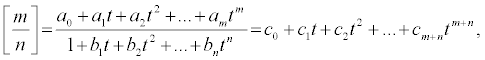(5)

Where ci’s are known coefficients and, ai’s and bi’s should be determined. The numerator and denominator have no factors in common.

#### Homogeneous Smoluchowski Coagulation Equation

The physical process of coagulation of particles is often modeled by Smoluchowski's equation. This equation is widely applied to describe the time evolution of the cluster-size distribution during aggregation processes. In this paper, the following Smoluchowski's equation [5,6], will be considered;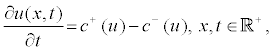(6)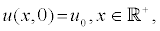(7)

Where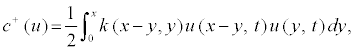(8)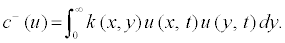(9)

u0, is a known function. u(x, t), is the density of cluster of mass x per unit volume at time t.

#### Applications

To illustrate the ability and the simplicity of the methods two examples are presented.

Example 1: Equations. (6)-(9) are considered with constant kernel, k (x, y)=1, and u0=exp (-x), [5-7]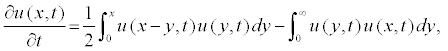(10)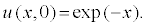(11)

The exact solution is,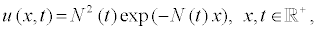(12)

With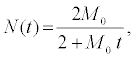To solve Equation (10), the following correction functional is constructed,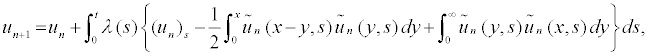(13)

where λ is general Lagrange multiplier,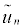is considered as restricted variations. To determine the value of λ the following procedure should be followed,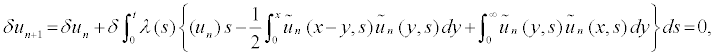(14)

which is equivalent to,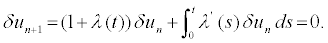(15)

Stationary conditions on Equation (15) lead to,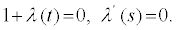(16)

The Lagrange multiplier is obtained as, λ (s)=−1. having this multiplier the iterative formula (13) turns to;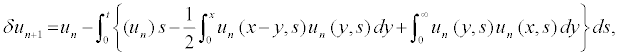(17)

Starting with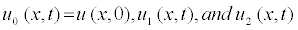are computed as follows;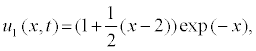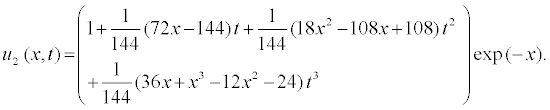Other approximations easily obtain by (17). The fifth-order iterative solution is considered as the approximate solution. Now, diagonal Pade approximation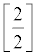is calculated as follows;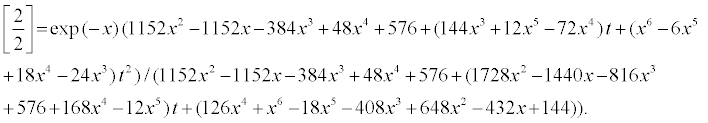(18)

Exact solution, approximate solutions via VIM, and VIM-Pade, and absolute errors of these two methods are plotted in Figures 1a-1k.

Figure 1: (a,b): Plots of example 1. (c): Plot of VIM-Pade approximation. (d): Plot of absolute error of VIMapproximation. (e): Plot of absolute error of VIM-Pade approximation. (f): VIM approximation at time. (g,h): At time VIM approximation at time. (i): Plot of absolute error of VIM-Pade approximation at time. (j): Plot of absolute error of VIM Pade approximation at time. (k): Plot of absolute error of VIM-Pade approximation at time.

Example 2: As the second example, Equations. (6)-(9) are considered with the multiplicative kernel,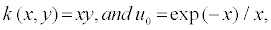, [5-7]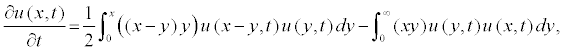(19)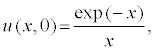(20)

with the exact solution,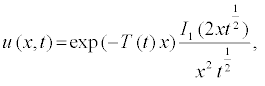Where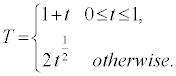And I1 is the modified Bessel function of the first kind,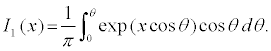Similar to previous example, Lagrange multiplier is determined as; λ (s)=−1 iterative relation may be constructed as follows,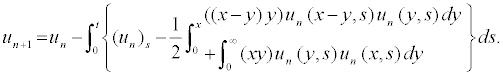(21)

Starting with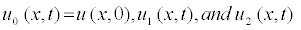are computed as follows;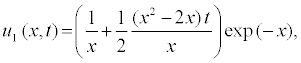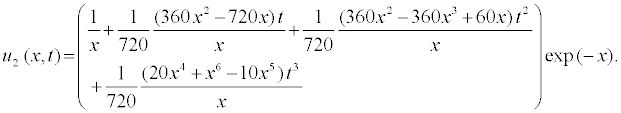Other approximations will be easily obtained by (21). The fourthorder iterative solution is considered as the approximate solution. Now, diagonal Pade approximationcan be calculated as follows;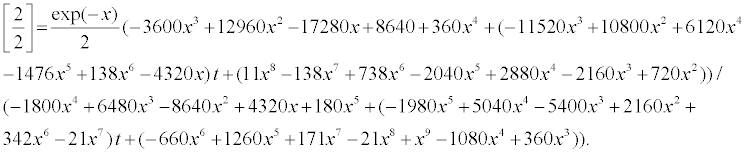(22)

Exact solution, VIM and VIM-Pade approximations, and errors of these two approximations are plotted in Figures 2a-2k.

Figure 2: (a): Plot of the exact solution of Eq. (19). (b): Plot of VIM approximation. (c): Plot of VIM-Pade approximation. (d): Plot of absolute error of VIM approximation at time. (e): Plot of absolute error of VIM-Pade approximation. (f): Plot of absolute error of VIMapproximation at time. (g): Plot of absolute error of VIM approximation at time. (h): Plot of absolute error of VIMapproximation at time. (i): Plot of absolute error of VIM-Pade approximation at time. (j): Plot of absolute error of VIM-Pade approximation at time. (k): Plot of absolute error of VIM-Pade approximation at time.

#### Conclusion

In this paper, Variational Iteration Method (VIM) and VIMPade have been employed to solve the homogeneous coagulation Smoluchowski equation. Obtained solutions have been compared with the exact solutions. Figures 1 and 2 show that the solutions of VIM and VIM-Pade are in good agreement with exact solutions. Also, for two examples the maximum absolute errors by using of VIM-Pade are lesser than those of VIM. Computations are performed by using the package Maple 13.

#### References

Select your language of interest to view the total content in your interested language

### Article Usage

• Total views: 12469
• [From(publication date):
July-2015 - Aug 23, 2019]
• Breakdown by view type
• HTML page views : 8652
• PDF downloads : 3817

## Post your commentCan't read the image? click here to refresh
###### Peer Reviewed Journals

Make the best use of Scientific Research and information from our 700 + peer reviewed, Open Access Journals

International Conferences 2019-20

Meet Inspiring Speakers and Experts at our 3000+ Global Annual Meetings

Top